# Introduction

The main objective of R-package VCA is to perform variance component analyses (VCA). VCA are a way to assess how the variability of a dependent variable is structured taking into account its association with one or multiple random-effects variables. Proportions of the total variability found to be attributed to these random effects variables are called variance components (VC). Thus, VCA is the procedure of estimating the amount of the VCs’ contribution to the total variability in the dependent variable. Moreover, there are methods provided for estimating confidence intervals (CI) of VCs along with different graphical tools to better understand the data and for detecting outliers. Also included, but usually of less importance in the field of VCA: Estimation of fixed effects and least square means (LS means) as well as testing linear hypotheses of fixed effects/LS means of linear mixed models (LMMs).

VCs can be predicted in random models (random effects or variance component models) and LMMs (linear mixed -effects- models) by application of either analysis of variance (ANOVA)-type estimation or Restricted Maximum Likelihood (REML). Experiments of this type frequently occur in performance evaluation analyses of diagnostic tests or analyzers (devices) quantifying various types of measurement (im)precision (see e.g. CLSI EP05-A3 guideline). In this setting it is important to point out that precision and imprecision both refer to the variability of measurements and differ only by their respective point of view, i.e. the larger the precision of a measuring method the smaller is its imprecision and vice versa.

In the course of the discussion of R-package VCA, several examples will be given to allow the user to better understand the application of the most important functions. For all of the examples, simulated data sets coming with the R-package will be used. One of these, VCAdata1, comprises 2520 observations. There are 6 variables for 3 devices (device), 3 lots (lot), 10 samples (sample), 21 days (day) and 2 runs within day (run) with 2 replicates per run. This means, for each run two measurements (y) were performed under conditions which are as constant as they can get. One commonly speaks of repeatability measurement conditions.

## 'data.frame':    2520 obs. of  6 variables:
##  $lot : Factor w/ 3 levels "1","2","3": 1 1 1 1 1 1 1 1 1 1 ... ##$ sample: Factor w/ 10 levels "1","2","3","4",..: 1 1 1 1 1 1 1 1 1 1 ...
##  $day : Factor w/ 21 levels "1","2","3","4",..: 1 1 1 1 2 2 2 2 3 3 ... ##$ run   : Factor w/ 2 levels "1","2": 1 1 2 2 1 1 2 2 1 1 ...
##  $y : num 2.11 2.09 2.73 2.72 2.87 ... ##$ device: Factor w/ 3 levels "1","2","3": 1 1 1 1 1 1 1 1 1 1 ...

For all other data sets used in this document please refer to the description that can be found in R-package VCA.

# Visualization of Variability via Function varPlot

Visualization can often help in understanding new or unknown data. When performing a VCA it is highly recommended to initially take a look at a variability chart to better understand the major sources of variability and to get a rough idea of the general total variability to expect. Moreover, the variability chart can help spotting abnormalities such as outliers in the data, i.e. extreme values that lie an abnormous distance from the other remaining values and therefore are likely to have a negative effect on the analysis and possible lead to invalid results (see sections Outlier Detection and Checking Normality and Extreme Values Using R-Package STB).

## Default Settings

VCA provides a function called varPlot for generating such a variability chart. To create a variability chart by varPlot(), it is necessary to state the model formula as well as the data set as function parameters. In this first example, a variability chart for a random model of the form y~(device+lot)/day/run will be plotted. All effects in a VCA are modeled as random since the interest lies in their distribution, i.e. their contribution to the total variability (variance). Run is nested within day, and day is, according to the model formula, nested within combinations of lot and device. Please note that in varPlot() the real model is not relevant but rather the order of the variables which determines the layout of the table depicted at the bottom of the variability chart. The implementation of the variability chart cannot distinguish between nested and crossed terms. The data that is used for drawing the variability chart is datS5, a subset of VCAdata1 consisting only of 252 observations from sample number 5 from a total of 10 samples:

library(VCA)
datS5 <- subset(VCAdata1, sample==5)

Given the parameters form and Data only, varPlot() creates the variability chart in a plain design:

varPlot(form=y~(device+lot)/day/run, Data=datS5)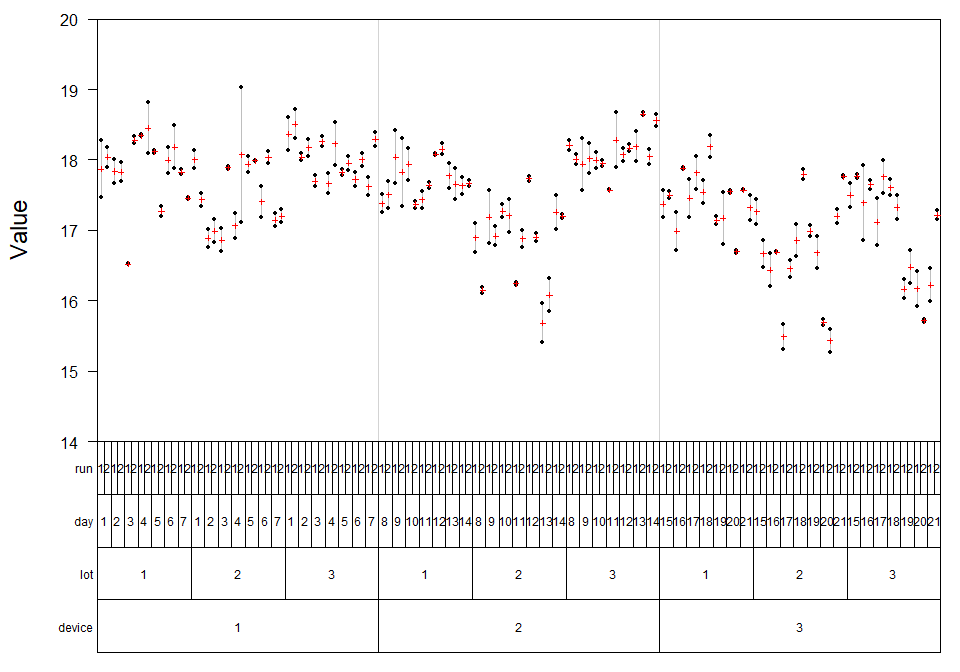The variability chart shows all 252 observations in datS5 according to the nesting structure of the model formula. Again, this equals 3 devices (bottom line) with 3 lots each (second from bottom), 7 measuring days (third from bottom) per lot and 2 runs per day. Since each run consists of two replicates, these two values are drawn and connected by a vertical line highlighting the complete range of replicated measurements. Whenever measurements are performed under identical conditions (replicated), i.e. no other sources of variability have an influence, the variance of residual error will be inferred from these differences. In the setting of in-vitro diagnostics one speaks of repeatability variance meaning the pure assay (im)precision.

It is possible to specify the display of further graphical elements in the variability chart using various style parameters to improve the overview and facilitate information extraction. Almost all parameters correspond to list-type objects. This allows very detailed specification of the graphical appearance, e.g. in the parameter MeanLine variables can be selected whose mean-values are to be highlighted as horizontal lines, their colors (col), line-width (lwd), line-type (lty) etc.. In fact, all function arguments accepted by the R-function lines can be specified, since the list MeanLine is passed forward to lines() with some modifications taking place beforehand.

In the next example, horizontal lines for mean-values (MeanLine) are drawn for the intercept as well as the factors device and lot. Via list-element col the appropriate colors are set to white, blue and magenta. Using list-element lwd allows to change the line width of the mean value horizontal lines. The allocation of style parameter settings to the respective graphical elements/variables is done in accordance with the order of element denomination. Furthermore, the three levels of variable lot will be highlighted in different shades of gray using function argument BG, which also needs to be specified as list:

varPlot(y~(device+lot)/day/run, datS5,
MeanLine=list(var=c("int", "device", "lot"),
col=c("white", "blue", "magenta"), lwd=c(2,2,2)),
BG=list(var="lot", col=paste0("gray", c(70,80,90))))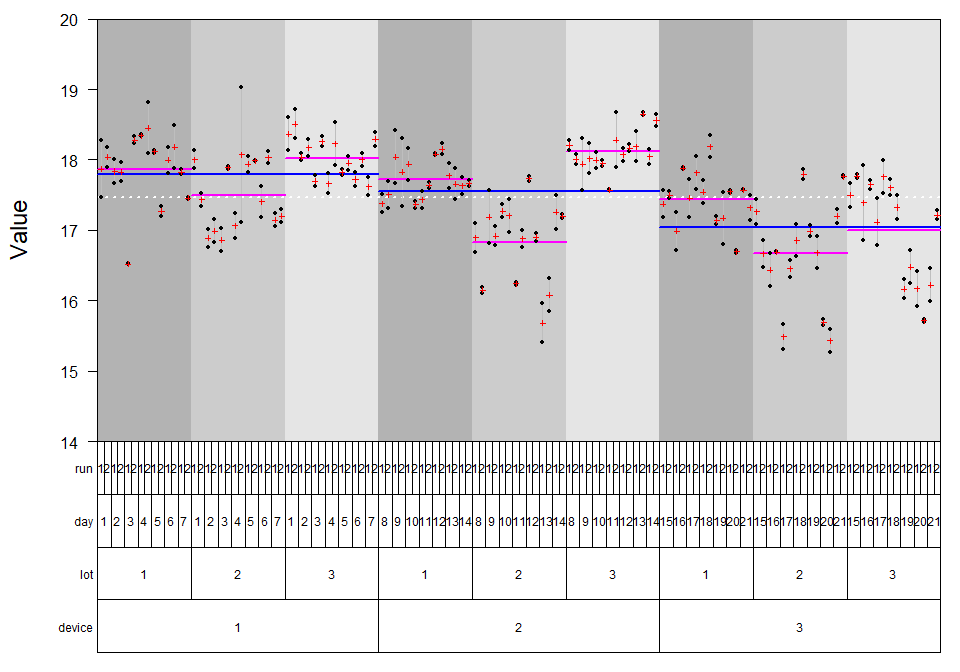# Outlier Detection

## Outlier Detection by Visual Inspection

From looking at the variability chart it is noticeable that there is one pair of measurements that deviates remarkably far from its within-run mean, i.e. the small red cross on the line connecting the values vertically. These two potential outliers are the replicates of run 2 on day 4, measured in lot 2 with device 1. Extreme values might negatively influence (violate) the normality assumption applied for all random variates of a linear mixed effects model (i.e. random effects, residuals). Thereby, outliers can influence the validity of the model and its results. Following commented R-code is used to generate the variability chart with the potential outliers highlighted:

#indicate outliers by new variable
datS5$out <- 0 datS5$out[which(datS5$device==1 & datS5$lot==2 & datS5$day==4 &datS5$run==2)] <- 1

varPlot(y~(device+lot)/day/run, datS5,
# plots horizontal lines for sub-sets
MeanLine=list(  var=c("int", "device", "lot"),
col=c("white", "blue", "magenta"),
lwd=c(3,3,3)),
# colors the background according to a variable's levels
# 'col.table=TRUE' indicates the variable in the table
BG=list(var="lot", col=paste0("gray", c(70,80,90)),
col.table=TRUE),
# tailoring the appearance of measurements (points)
Points=list(pch=list(var="out", pch=c(21, 24)),
col=list(var="out", col=c("black", "red")),
bg= list(var="out", bg=c("white", "yellow")),
cex=list(var="out", cex=c(1, 1.5))),
# specification of the text within the table below
VarLab=list(list(cex=1.75), list(cex=1.5),
list(cex=1, srt=90), list()),
# variable names right of the table (since 'side=4')
VCnam=list(cex=1.25, side=4),
# use 33% of the height of the upper part for the table
htab=.33)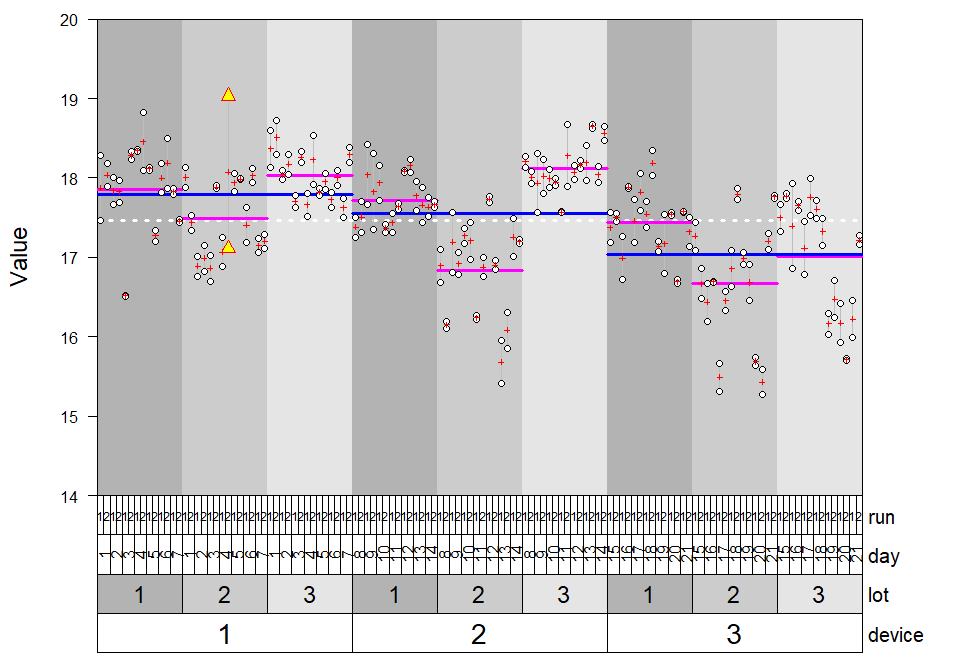The function argument Points can also be used as list of arguments passed forward to function points(). But it can be used in a more detailed manner letting the user specify list-elements pch, col, bg, and cex as lists themselves. By doing so one can incorporate additional information indicated by different plotting symbols, color, and size of the symbols. Element bg is only useful in case of setting pch equal to 21-25. Then, col is interpreted as the color of the border and bg as the background color of these symbols (see also the last example of the varPlot help).

## Outlier Detection Using Studentized Residuals

Studentization of residuals and/or random effects transforms these random variates to have mean zero and standard deviation (SD) equal to 1 using their element-wise variance estimates for standardization. Outliers amongst studentized conditional residuals correspond to outliers on the replicate level, i.e. where all measuring conditions are kept as constant as possible (here: device, lot, day and run). Studentized conditional residuals can be assessed and plotted by the VCA-function plotRandVar. plotRandVar() requires a fitted model as input. In addition, the parameter term specifies the type of residuals whereas the parameter mode specifies the transformation to be applied to the residuals.

There are two options available for fitting a variance component model. One can use either ANOVA-type estimation via function anovaVCA or REML-estimation via function remlVCA. For balanced data and in situations where ANOVA-estimation does not produce negative variance-estimates, both methods generate identical results. Otherwise, both approaches to estimation of random effects, and therefore VCs, are likely to differ. Although this difference is usually small. We are using ANOVA-type estimation here but all statements are also valid for REML-estimation. Note that there is function fitVCA wrapping function calls to anovaVCA and remlVCA.

Fitting the model to the data returns the object fitS5 of class VCA:

fitS5 <- anovaVCA(y~(device+lot)/day/run, datS5)
# if varPlot was called before, better shut down the old graphics device
# graphical parameters were not reset to allow the user to add further
# information to the variability chart, which would not be possible otherwise
dev.off()
plotRandVar(fitS5, term="cond", mode="student")

Residuals that deviate further than 3 times the standard deviation can be considered as extreme, since the expected value of the mean after studentization is equal to 1. Thus, only 0.3% of all observations are expected to be outside of the $$+/- 3 \times SD$$ interval. There are two values that come into question as can be seen in the plot drawn by plotRandVar():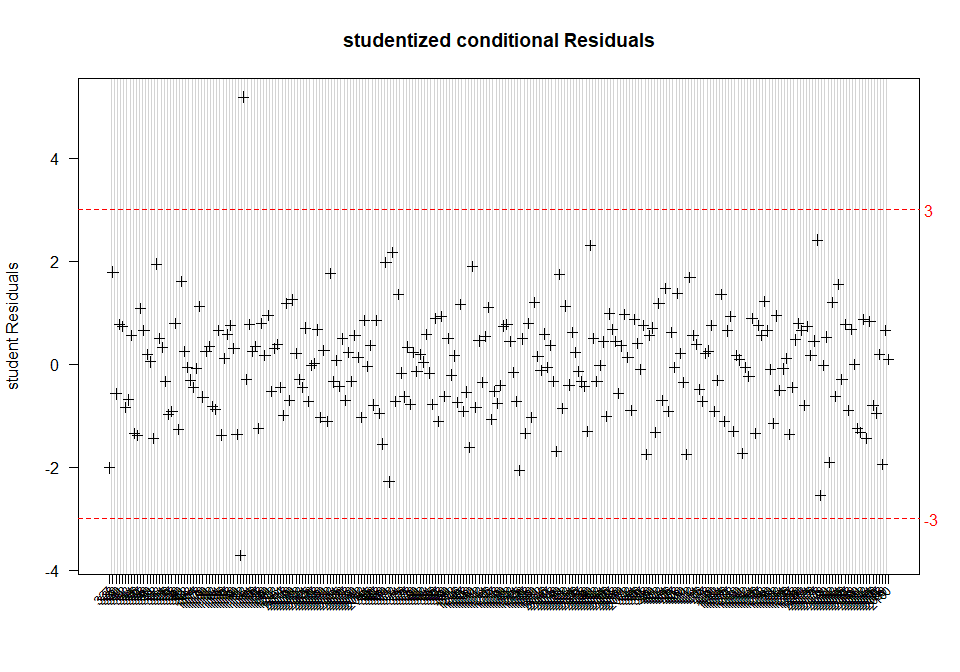However, since there are too many values, the labelling of the x-axis has become unclear. Setting the function’s parameter pick to TRUE allows selecting specific residuals by clicking on them within the graphics device window:

plotRandVar(fitS5, term="cond", mode="student", pick=TRUE)
abline(h=c(-3, 3), lty=2, col="red", lwd=2)
mtext(side=4, at=c(-3, 3), col="red", line=.25, las=1, text=c(-3, 3))

For every value selected in the plot the respective row name within the data set will be displayed next to the value: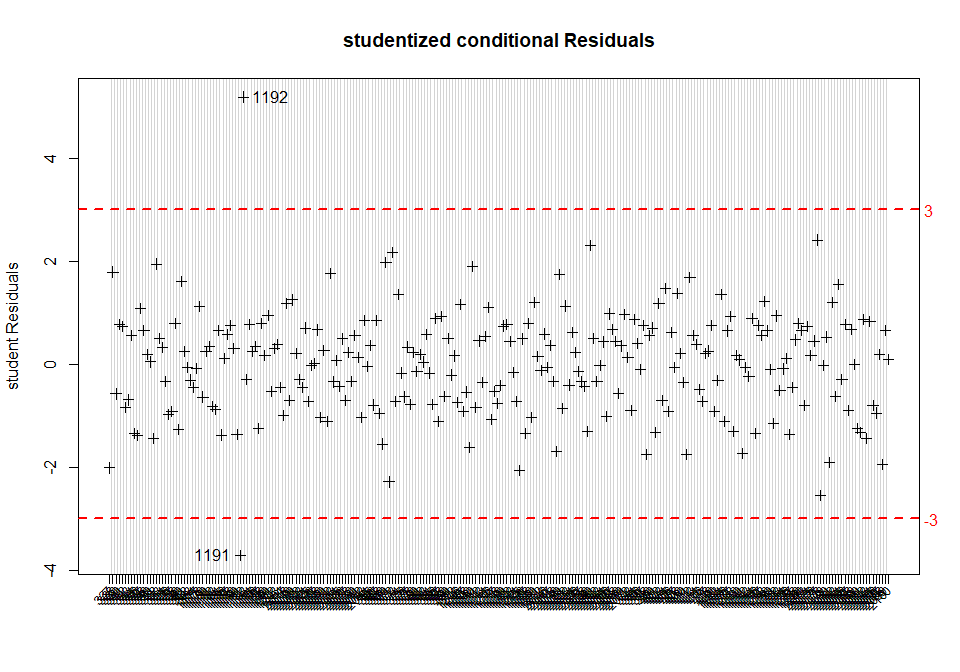Please note: After having selected the desired data points, the graphics procedure has to be stopped manually by right-clicking on the graphics device output and selecting “Stop” in order to be able to execute further arguments within the R-console.

To double-check whether the manually picked data points really are the replicate measurements initially verified from sighting the variability chart, simply select these observations using their row names:

datS5[c("1191","1192"),]
##      lot sample day run     y device out
## 1191   2      5   4   2 17.11      1   1
## 1192   2      5   4   2 19.03      1   1

In fact, these observations are the ones initially suspected. The values should be considered for exclusion from the data set to prevent invalid results. See Checking Normality and Extreme Values Using R-Package STB for further methods that help determine whether these observations really have to be excluded in view of the normality assumption.

## An Outlier Detection Algorithm

Extreme values on the replicate-level are supposed to be detected by means of the Grubbs-test (CLSI EP05-A3). A modified version of Grubbs-test may be defined by means of the median and the MD68-statistic. The median can be seen as the robust version of the mean which is known to be sensitive to outliers, thus itself biased in case real outlying observations exist. The MD68 can be seen as the robust version of the SD, defined as follows:

# Function computing the MD68 using SAS PCTLDEF5 quantile definition.
# x (numeric) values from which the MD68 shall be computed
# na.rm (logical) TRUE = missing values will be excluded automatically
md68 <- function(x, na.rm=FALSE)
{
stopifnot(is.numeric(x))
Med     <- median(x, na.rm=na.rm)
Diff    <- abs(x-Med)
MD68    <- quantile(Diff, probs=.68, type=2)
MD68
}

The critical value for this robust version of the MD68-based outlier detection algorithm was found to be reasonably well working when set equal to $$2.75 \times MD68$$. Specifically, the deviation on the replicate-level from the median of the respective replicate group may not exceed a $$2.75 \times MD68$$. The replicate groups in our running example are always within run as the smallest grouping-variable where constant measuring conditions can be assumed. The application of this outlier-detection algorithm should be done in groups where so called intermediate precision measuring conditions exist. In the example this refers to a single lot on a single device, i.e. nine such groups exist here (3 devices x 3 lots). For each of these nine groups the specific MD68-estimate should be computed and the observation-specific deviation from the median of the replicate group should be compared to the critical value of $$2.75 \times MD68$$.

# identify groups of intermediate precision (IP) measuring conditions
datS5$IPgroup <- paste(datS5$device, datS5$lot, sep="_") # uniquely identify replicate groups within IP-groups datS5$RepGroup <- paste(datS5$day, datS5$run, sep="_")

# Define a function performing the MD68-based outlier-algorithm.
# The data.frame will be returned with two additional variables,
# "diff" (absolute differences from the replicate-group median) and
# "outlier" (TRUE = outlier, FALSE = no outlier).
#
# obj       (data.frame) with at least two variables
# resp      (character) name of the numeric response variable
# RepGroup  (character) name of the replicate-grouping variable

md68OutlierDetection <- function(obj=NULL, resp=NULL, RepGroup=NULL)
{
stopifnot(is.data.frame(obj))
cn      <- colnames(obj)
stopifnot(is.character(resp) && resp %in% cn && is.numeric(obj[,resp]))
stopifnot(is.character(RepGroup) && RepGroup %in% cn)
MD68    <- md68(obj[, resp])
Crit    <- MD68*2.75
# tapply returns a list with as many elements as there are unique
# elements in obj[,RepGroup], which must be converted to a vector
obj$diff <- unlist( tapply(obj[,resp], obj[,RepGroup], function(x) { m <- median(x) d <- abs(x - m) d })) obj$threshold   <- Crit
obj$outlier <- obj$diff > Crit
obj
}

To apply this MD68-based outlier detection algorithm to the data, IP-group by IP-group, one can use a simple for-loop. The result is data.frame out which has three additional variables (threshold, diff, and outlier).

IPgroups <- unique(datS5$IPgroup) for(i in 1:length(IPgroups)) { tmpData <- subset(datS5, IPgroup == IPgroups[i]) if(i == 1) out <- md68OutlierDetection(tmpData, resp="y", RepGroup="RepGroup") else out <- rbind(out, md68OutlierDetection(tmpData, resp="y", RepGroup="RepGroup")) } head(out) ## lot sample day run y device out IPgroup RepGroup diff threshold ## 337 1 5 1 1 17.46 1 0 1_1 1_1 0.410 1.252 ## 338 1 5 1 1 18.28 1 0 1_1 1_1 0.410 1.252 ## 339 1 5 1 2 17.89 1 0 1_1 1_2 0.145 1.252 ## 340 1 5 1 2 18.18 1 0 1_1 1_2 0.145 1.252 ## 341 1 5 2 1 18.01 1 0 1_1 2_1 0.170 1.252 ## 342 1 5 2 1 17.67 1 0 1_1 2_1 0.170 1.252 ## outlier ## 337 FALSE ## 338 FALSE ## 339 FALSE ## 340 FALSE ## 341 FALSE ## 342 FALSE # Were there any outliers detected? any(out$outlier)
##  FALSE

The expression shown above states that no element of variable outlier takes the value TRUE, i.e. no outlier was identified. As one can see by thoroughly inspecting the R source-code, for these data the outlier detection algorithm seems to suffer from relatively large between-day and between-run variability. Both contribute to the total variance within IP-group 1_2 additionally to the measurement imprecision, which is reflected in a rather large threshold value $$2.75 \times MD68 = 1.624$$. In consequence, this results in not detecting the previously identified two observations 1191 and 1192 which deviate substantially from each other under repeatability conditions. The above outlined extreme value detection is thus rather conservative, i.e. replicates have to differ a lot in order to be termed extreme.

## Exteme Values on all Levels

Outliers may occur on all levels in an LMM, other levels than replicates should be assessed using studentized random effects. This is possible by specifying the desired random effects term in the term parameter of plotRandVar(). The exact term denomination can be obtained from the ANOVA-table visible when printing the VCA-object. It is also part of the VCA-object fitS5 :

# use non-default number of decimal places
print(fitS5, digits=4)
##
##
## Result Variance Component Analysis:
## -----------------------------------
##
##   Name               DF      SS      MS      VC     %Total  SD     CV[%]
## 1 total              15.1786                 0.6145 100     0.7839 4.4889
## 2 device             2       24.8695 12.4348 0.1379 22.4425 0.3714 2.1266
## 3 lot                2       27.2843 13.6421 0.1523 24.7816 0.3902 2.2346
## 4 device:lot:day     58      49.3208 0.8504  0.1403 22.8281 0.3745 2.1448
## 5 device:lot:day:run 63      18.2223 0.2892  0.1052 17.1217 0.3244 1.8574
## 6 error              126     9.9308  0.0788  0.0788 12.8261 0.2807 1.6076
##
## Mean: 17.46 (N = 252)
##
## Experimental Design: balanced  |  Method: ANOVA

As an example, consider the studentized random effects of “device:lot:day”. This reveals the between-day variability:

plotRandVar(fitS5, term="device:lot:day", mode="student")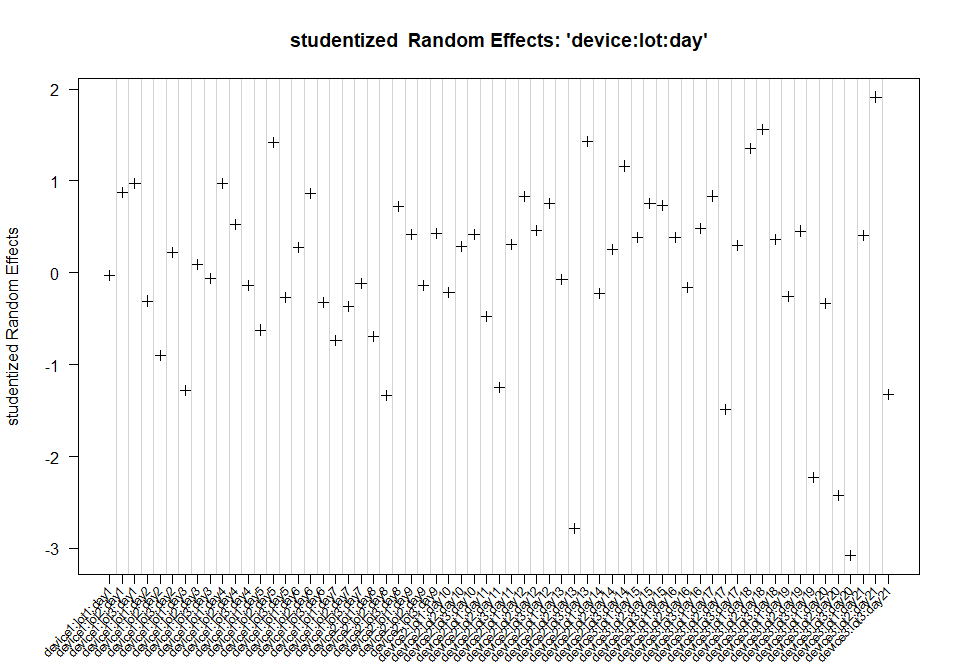As well as the studentized random effects of “device:lot:day:run”, which show the within-run variability:

plotRandVar(fitS5, term="device:lot:day:run", mode="student")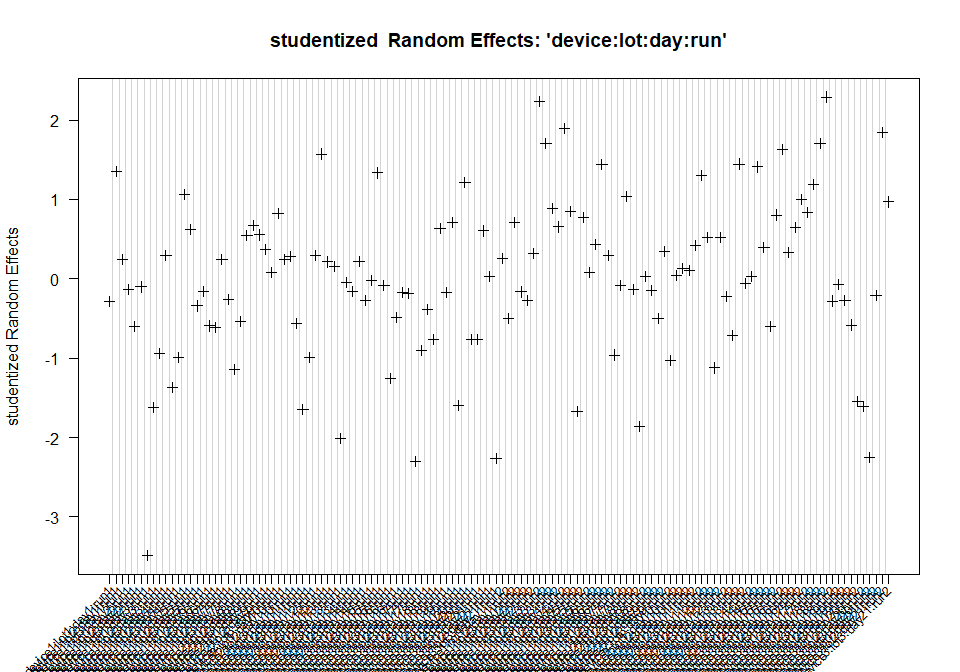Please note: If there are too few factor levels (here e.g. for device and lot with 3 levels each), the assessment of studentized random effects is only of limited use.

fitS5\$aov.tab
##                        DF     SS       MS      VC %Total     SD CV[%]
## total               15.18     NA       NA 0.61450 100.00 0.7839 4.489
## device               2.00 24.870 12.43476 0.13791  22.44 0.3714 2.127
## lot                  2.00 27.284 13.64213 0.15228  24.78 0.3902 2.235
## device:lot:day      58.00 49.321  0.85036 0.14028  22.83 0.3745 2.145
## device:lot:day:run  63.00 18.222  0.28924 0.10521  17.12 0.3244 1.857
## error              126.00  9.931  0.07882 0.07882  12.83 0.2807 1.608

# Checking for Normality and Extreme Values Using R-Package STB

As indicated before in section Outlier Detection, it is assumed that random factors are stochastically independent and random effects are normally distributed. However, extreme values, i.e. outliers, can violate this normality assumption and therefore distort estimations. The rule of thumb is to exclude as few observations as possible and as many as necessary, i.e. generally a maximum of two outliers that violate the normality assumptions at most.

A reasonable means to visually check for observations or extreme values that violate this assumption is to draw a Q-Q plot (quantile-quantile plot). In this context, the Q-Q plot computes the theoretically expected random variates (i.e. random effects or residuals) given a normal distribution. This results in a straight diagonal line. Then the observed random variate values are drawn against the expected values. If the observed values evidently deviate from the straight diagonal line, the data most likely are not normally distributed. An additional aid in visually determining if observations violate the normal assumption is to add tolerance bands to the Q-Q plot.

R-package STB (https://CRAN.R-project.org/package=STB) provides function stb.VCA which simulates Ndata sets incorporating the variance- covariance structure of a fitted object of class VCA and constructs a 100(1-alpha)% simultaneous tolerance band (STB) for the simulated data. The STB is based on random variates exctracted from the N simulated data sets. The construction of the tolerance band requires a reasonably large number of simulations to calculate sufficiently exact STB (see Schuetzenmeister and Piepho 2012). In the following example, simultaneous tolerance bands from studentized (mode) conditional (term) residuals extracted from 5000 simulated data sets (N) are created using method stb for objects of class VCA. To do so, an LMM fit for datS5 is required. By way of example, all model terms are assumed to be random:

set.seed(23)
fitS5.LMM <- anovaMM(y~((device)+(lot))/(day)/(run), datS5)
STB.res <- stb(fitS5.LMM, term="cond", mode="student", N=5000)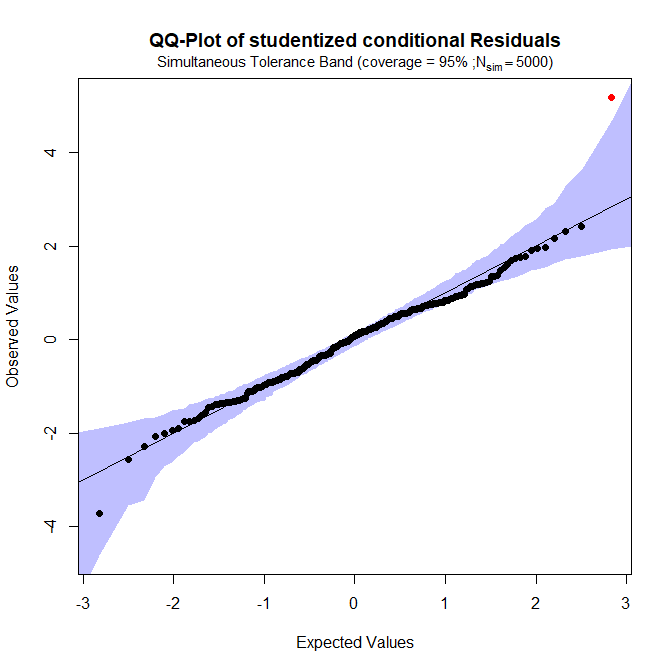One can clearly see that there is one observation in the top right corner lying outside of the tolerance band. Applying the generic R-function plot to the object STB.res created above and setting its parameter pick=TRUE will allow for manual selection of observations by clicking on them within the graphics device window - just as shown in the example above when discussing the variability chart:

plot(STB.res, pick=TRUE)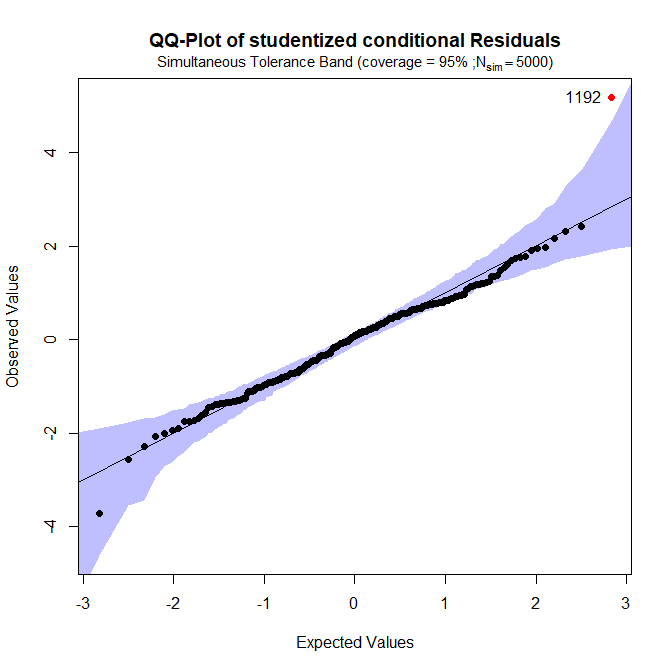Since observation 1192 is violating the normality assumption, it is removed from the original data set and the STB is calculated again. First a VCA-object has to be generated fitting a model, here, using function anovaMM designed to fit linear mixed models. Terms intented to be modeled as random have to be enclosed in parantheses indicating this:

datS5.reduced <- datS5[!rownames(datS5) == "1192",]
fitS5.reduced <- anovaMM(y~((device)+(lot))/(day)/(run), datS5.reduced)
STB.res2 <- stb(fitS5.reduced, term="cond", mode="student", N=5000)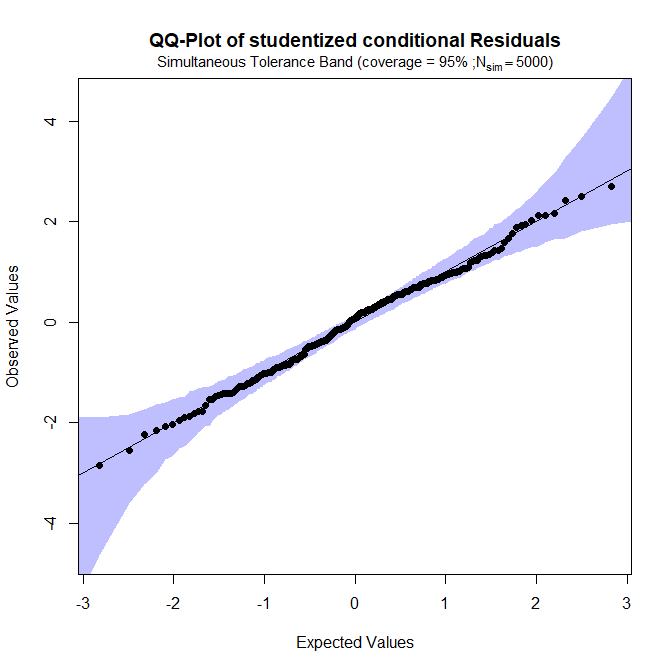As it turns out, removing only the observation 1192 already results in a QQ-plot that obviously does not show any indications for the violation of the normality assumption anymore. To double check another plot of studentized conditional residuals is created from the fitted model of the reduced data set:

plotRandVar(fitS5.reduced, term="cond", mode="student")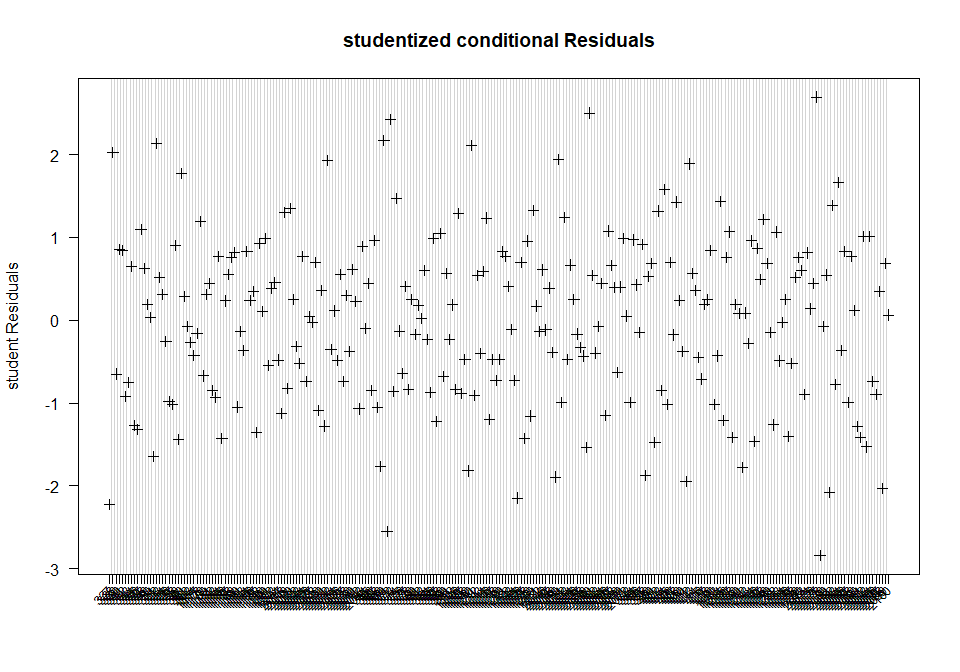In fact, observation 1191 of the initially (see section Outlier Detection) suspected value pair does not have to be excluded from the data set anymore.

Excluding too many observations potentially leads to inaccurate estimates since information is lost. On the other hand, not excluding observations that should be removed from the data because of violating the normality assumption leads to invalid estimates. Once again, the following ANOVA table shows the estimates that result from fitting the original full data set datS5 still containing observation 1191 and 1192. Pay special attention to the error’s contribution to the total variability (see %Total column):

##                        DF     SS       MS      VC %Total     SD CV[%]
## total               15.18     NA       NA 0.61450 100.00 0.7839 4.489
## device               2.00 24.870 12.43476 0.13791  22.44 0.3714 2.127
## lot                  2.00 27.284 13.64213 0.15228  24.78 0.3902 2.235
## device:lot:day      58.00 49.321  0.85036 0.14028  22.83 0.3745 2.145
## device:lot:day:run  63.00 18.222  0.28924 0.10521  17.12 0.3244 1.857
## error              126.00  9.931  0.07882 0.07882  12.83 0.2807 1.608

Removing only observation 1192, since it caused the violation of the normality assumption, results in the following estimates. Note how the error %Total reduced from 12.83% before to 10.64% now:

##                        DF     SS      MS     VC %Total     SD CV[%]
## total               14.41     NA      NA 0.6081 100.00 0.7798 4.467
## device               2.00 23.952 11.9759 0.1330  21.87 0.3647 2.089
## lot                  2.00 28.693 14.3464 0.1613  26.53 0.4017 2.301
## device:lot:day      58.00 49.211  0.8485 0.1443  23.72 0.3798 2.176
## device:lot:day:run  63.00 17.212  0.2732 0.1048  17.24 0.3237 1.854
## error              125.00  8.088  0.0647 0.0647  10.64 0.2544 1.457

# Commonly Used VCA-Models

In this section we want to give an overview on the most commonly used VCA-models. The CLSI EP05-A3 guideline describes multiple experimental settings, e.g. the single-site evaluation study and the multi-site evaluation study. Here, we show how these experiments can be analyzed using R-package VCA.

## $$20 \times 2 \times 2$$ Single Site Evaluation

The single site experiment recommended in the CLSI EP05-A3 consists of 20 days, 2 runs per day with 2 replicates per run ($$20 \times 2 \times 2 = 80$$).

# Function converts a color-string into RGB-code
# col (character) string specifying an R-color
# alpha (numeric) degree of transparency in [0, 1], 0=fully transparency, 1=opaque
asRGB <- function(col, alpha)
rgb(t(col2rgb(col))/255, alpha=alpha)

data(dataEP05A2_3)

varPlot(y~day/run, dataEP05A2_3,
# controls horizontal mean lines
MeanLine=list(var=c("int", "day"), col=c("gray75", "blue"), lwd=c(2,2)),
# controls how points (concentrations) are plotted, here using semi-transparency
# to see overlayed points
Points=list(pch=16, col=asRGB("black", .5), cex=1.25),
# controls how replicate-means are plotted
Mean=list(col="magenta", cex=1.25, lwd=2),
# controls how the title is shown
Title=list(main="20 x 2 x 2 Single-Site Evaluation", cex.main=1.75),
# controls plotting of levels per VC, if as many lists as there are VCs are
# specified, each VC can be specified individually
VarLab=list(list(cex=1.5), list(cex=1.25)),
# controls how names of VCs are plotted
VCnam=list(font=2, cex=1.5),
# controls appearance of the Y-axis label
YLabel=list(text="Concentation [mg/dL]", las=0, line=3, font=2, cex=1.25),
# Y-axis labels rotated
las=1)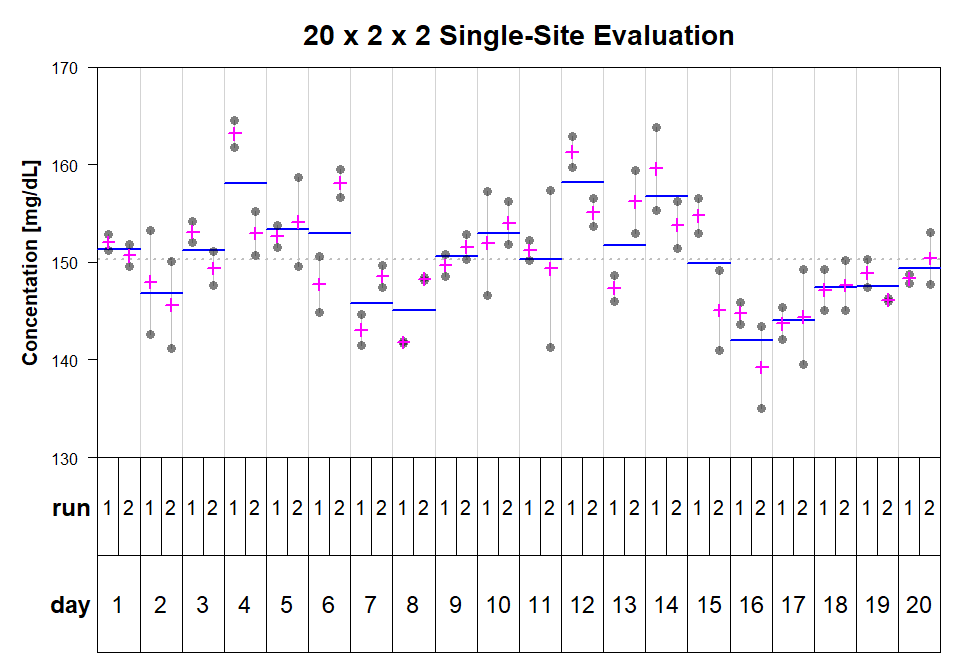The VCA package comes with three example data sets of this type (dataEP05A2_1, dataEP05A2_3, dataEP05A2_3). Here, run is nested within day, since all 20 days are independent from each other, thus, run No. 1 on a given day does not have anything in common with run No. 1 on another day. This can be expressed as shown below using the nesting-operator ‘/’. After the model was fitted to the data some additional inferential statistics are usually of interest, such as confidence intervals (CI) for VCs and/or performing $$\chi^2$$-tests for claims of repeatability or total imprecision. This can be addressed using function VCAinference.

# fit 20 x 2 x 2 model to data
fit.SS3 <- fitVCA(y~day/run, dataEP05A2_3)
fit.SS3
##
##
## Result Variance Component Analysis:
## -----------------------------------
##
##   Name    DF        SS          MS        VC        %Total    SD       CV[%]
## 1 total   50.129098                       35.546313 100       5.962073 3.967511
## 2 day     19        1506.145222 79.270801 12.231099 34.40891  3.497299 2.327307
## 3 day:run 20        606.928138  30.346407 7.031193  19.780372 2.65164  1.764556
## 4 error   40        651.360839  16.284021 16.284021 45.810718 4.035346 2.685355
##
## Mean: 150.3 (N = 80)
##
## Experimental Design: balanced  |  Method: ANOVA
# estimate 95% confidence intervals, request CI for
# all variance components via 'VarVC=TRUE'
inf.SS3 <- VCAinference(fit.SS3, VarVC=TRUE)
inf.SS3
##
##
##
## Inference from (V)ariance (C)omponent (A)nalysis
## ------------------------------------------------
##
## > VCA Result:
## -------------
##
##   Name    DF      SS        MS      VC      %Total  SD     CV[%]  Var(VC)
## 1 total   50.1291                   35.5463 100     5.9621 3.9675
## 2 day     19      1506.1452 79.2708 12.2311 34.4089 3.4973 2.3273 47.0968
## 3 day:run 20      606.9281  30.3464 7.0312  19.7804 2.6516 1.7646 26.3372
## 4 error   40      651.3608  16.284  16.284  45.8107 4.0353 2.6854 13.2585
##
## Mean: 150.3 (N = 80)
##
## Experimental Design: balanced  |  Method: ANOVA
##
##
## > VC:
## -----
##         Estimate CI LCL  CI UCL  One-Sided LCL One-Sided UCL
## total   35.5463  24.8957 54.8935 26.338        51.0984
## day     12.2311  0*      25.6818 0.9429        23.5193
## day:run 7.0312   0*      17.0897 0*            15.4726
## error   16.284   10.9764 26.659  11.6818       24.571
##
## > SD:
## -----
##         Estimate CI LCL CI UCL One-Sided LCL One-Sided UCL
## total   5.9621   4.9896 7.409  5.1321        7.1483
## day     3.4973   0*     5.0677 0.9711        4.8497
## day:run 2.6516   0*     4.134  0*            3.9335
## error   4.0353   3.3131 5.1632 3.4179        4.9569
##
## > CV[%]:
## --------
##         Estimate CI LCL CI UCL One-Sided LCL One-Sided UCL
## total   3.9675   3.3203 4.9304 3.4152        4.7569
## day     2.3273   0*     3.3724 0.6462        3.2273
## day:run 1.7646   0*     2.751  0*            2.6176
## error   2.6854   2.2047 3.4359 2.2744        3.2986
##
##
## 95% Confidence Level  | * CI-limits constrained to be >= 0
## SAS PROC MIXED method used for computing CIs

The default-setting of function anovaVCA sets all negative variance estimates equal to zero, since negative values for variances are not defined. This is a known problem of ANOVA-estimation for variance components. There are several reasons which might cause negative variance estimates, e.g. the model specified does not match the structure of the data (wrong model) or there might be outlying observations negatively influencing model assumptions (normality) or the variability might just be too large. These explanation are given in SAS Documentation of PROC VARCOMP, in section Negative Variance Component Estimates.

## $$3 \times 5 \times 1 \times 5$$ Multi-Site Evaluation

The next model we would like to look at is used in the $$3 \times 5 \times 1 \times 5$$ multi-site evaluation study. Here, 3 devices (site, laboratories, . . . ) are used and each sample is measured on 3 days in a single run in 5 replicates resulting in 75 observations overall. R-package VCA comes with 3 such data sets (dataEP05A3_MS_1, dataEP05A3_MS_3, dataEP05A3_MS_3). This model assumes a single reagent-lot to be used on all three sites (devices, labs, . . . ). A nice-looking variability-chart for such data can be generated as follows:

data(dataEP05A3_MS_1)
varPlot(y~site/day, dataEP05A3_MS_1,
BG=list(var="site", col=paste0("gray", c(100, 80, 60))),
Points=list(pch=16, col=asRGB("black", .5), cex=1.25),
MeanLine=list(var=c("int", "site"), col=c("black", "orange"), lwd=c(2,2)),
Mean=list(col="cyan", cex=1.25, lwd=2), las=1,
YLabel=list(text="Concentation [mg/dL]", las=0, line=3, font=2, cex=1.25),
Title=list(main="Multi-Site Evaluation on dataEP05A3_MS_1", cex.main=1.75),
VCnam=list(font=2, cex=1.5),
VarLab=list(list(cex=1.5, font=2), list(cex=1.25, font=2)))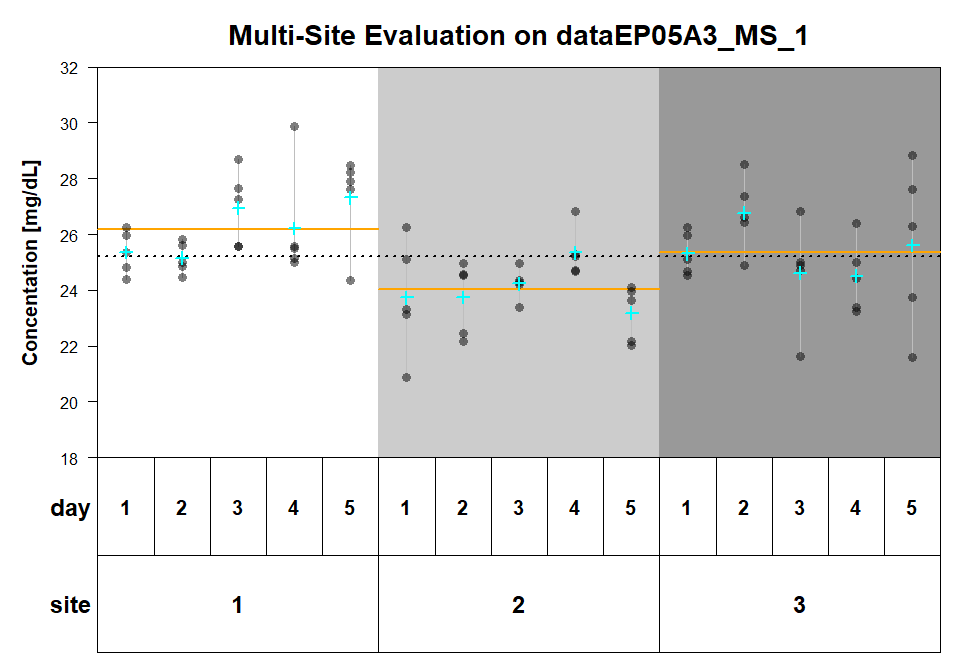The model itself can be fitted to the data using following code (now using REML).

# fit 3 x 5 x 1 x 5 model to data
fit.MS1 <- fitVCA(y~site/day, dataEP05A3_MS_1, method="REML")
fit.MS1
##
##
## Result Variance Component Analysis:
## -----------------------------------
##
##   Name     DF        VC       %Total    SD       CV[%]    Var(VC)
## 1 total    16.889879 3.635232 100       1.906629 7.566293 1.564832
## 2 site     1.488641  1.017401 27.987245 1.008663 4.002794 1.390671
## 3 site:day 2.105837  0.345882 9.514705  0.588117 2.333892 0.113621
## 4 error    60        2.271949 62.49805  1.507299 5.981587 0.172058
##
## Mean: 25.2 (N = 75)
##
## Experimental Design: balanced  |  Method: REML

## $$3 \times 5 \times 2 \times 3$$ Multi-Site Evaluation

The CLSI EP05-A3 guideline describes a second type of multi-site evaluation which takes into account variability betweenruns additionally. This model requires 15 additional observations compared to the $$3 \times 5 \times 2 \times 3$$model and is the $$3 \times 5 \times 2 \times 3$$ model. Thus, there are 2 runs per day with 3 replicates each resulting in 90 observations overall. Since there is no such data set contained in R-package VCA we will simulate such data, plot it and fit the respective model.

# simulate fit 3 x 5 x 2 x 3 model to data
set.seed(23)
dat.MS2 <- data.frame(  y=50 +
# 3 random effects for sites
rep(rnorm(3,0,2.5), rep(30, 3)) +
# 15 random effects for days
rep(rnorm(15,0, 2), rep(6, 15)) +
# 30 random effects for runs
rep(rnorm(30,0, 1), rep(3, 30)) +
# residual error (repeatability)
rnorm(90,0,1.5),
site = gl(3, 30, labels=paste0("Site_", 1:3)),
day = gl(5, 6, 90),
run =gl(2, 3, 90)
)

Now visualize this data set.

varPlot(y~site/day/run, dat.MS2,
BG=list(var="site", col=paste0("gray", c(100, 80, 60))),
Points=list(pch=16, col=asRGB("black", .5), cex=1.25),
MeanLine=list( var=c("int", "site", "day"),
col=c("black", "orange", "blue"),
lwd=c(2,2,2)),
Mean=list(col="cyan", cex=1.25, lwd=2), las=1,
YLabel=list(text="Concentation [mg/dL]", las=0, line=3, font=2, cex=1.25),
Title=list(main="3 x 5 x 2 x 3 Multi-Site Evaluation", cex.main=1.75),
VCnam=list(font=2, cex=1.5),
# controls for which variable vertical lines are added between levels
# and how these are plotted
VLine=list(var="day", col="gray75"),
VarLab=list(list(cex=1.5), list(cex=1.25), list(cex=1.25)))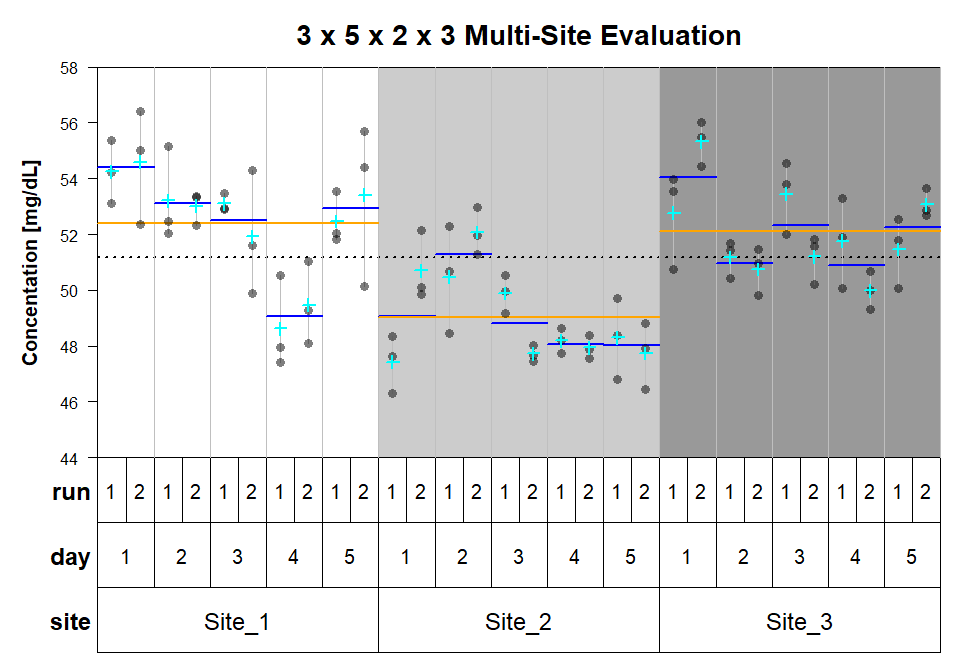And finally fit the respective VCA-model using ANOVA-type estimation.

# fit 3 x 5 x 2 x 3 model to data (ANOVA is default)
fit.MS2 <- fitVCA(y~site/day/run, dat.MS2)
print(fit.MS2, digits=4)
##
##
## Result Variance Component Analysis:
## -----------------------------------
##
##   Name         DF     SS       MS       VC     %Total  SD     CV[%]
## 1 total        8.2851                   7.2503 100     2.6926 5.2611
## 2 site         2      207.2225 103.6113 2.956  40.7713 1.7193 3.3594
## 3 site:day     12     179.1583 14.9299  1.837  25.3373 1.3554 2.6483
## 4 site:day:run 15     58.615   3.9077   0.7252 10.0026 0.8516 1.6639
## 5 error        60     103.9207 1.732    1.732  23.8888 1.3161 2.5714
##
## Mean: 51.18 (N = 90)
##
## Experimental Design: balanced  |  Method: ANOVA

## Multi-Site Multi-Lot Evaluation

The last model we want to address is the multi-lot reproducibility model. This refers to an experimental setup, where one of the two multi-site model is used with the additional variable reagent-lot. In in-vitro diagnostics reagent-lot is a factor where only a limited number of levels is available. Therefore, the typical multi-lot reproducibility design consists of 3 different reagent-lots used on 3 different sites. Different allocation schemes exist for assigning the 3 lots to the 3 sites. The best, but also the least parsimonious, design has all lots tested on all sites. These, among others, are valid lab-lot assignment schemes allowing to estimates VC reagent-lot:

##              Site_1 Site_2 Site_3
## ReagentLot_1 X      X      X
## ReagentLot_2 X      X      X
## ReagentLot_3 X      X      X
##              Site_1 Site_2 Site_3
## ReagentLot_1 X      X
## ReagentLot_2        X      X
## ReagentLot_3 X             X
##              Site_1 Site_2 Site_3
## ReagentLot_1 X      X
## ReagentLot_2        X      X
## ReagentLot_3 X             X

The original example data set VCAdata1 is very similar to the experimental designs described here. The sub-set datS5 refers to an experimental design with 3 site (device), 3 reagent-lots tested on each site, 7 days per site-lot combination, and 2 runs per day with 2 replicates per run.

In previous examples in this section we could always use a fully-nested model, because the testing-days in different laboratories are independent from each other, despite the fact that they might be encoded by integers 1 to 5. The same holds for runs, 2 runs performed on 2 different days are independent from each other despite the fact that both might be encoded using the same factor-level. This independence does not hold for reagent-lots. The same lots are used in all laboratories, each lot showing lot-specific performance, i.e. each lot will have a lot-specific bias compared to the unknown true concentration of a sample. This lot-specific bias directly translates to random effects for variable lot and their variation is modeled as following a normal distribution. At least this is assumed when estimation takes place. To reflect this in the model formula site and lot have to modeled as main-effects, and all other terms are nested within combinations of all main-effects. This can be done using following expressions.

fit.MSML <- fitVCA(y~(device+lot)/day/run, datS5)
print(fit.MSML, digits=4)
##
##
## Result Variance Component Analysis:
## -----------------------------------
##
##   Name               DF      SS      MS      VC     %Total  SD     CV[%]
## 1 total              15.1786                 0.6145 100     0.7839 4.4889
## 2 device             2       24.8695 12.4348 0.1379 22.4425 0.3714 2.1266
## 3 lot                2       27.2843 13.6421 0.1523 24.7816 0.3902 2.2346
## 4 device:lot:day     58      49.3208 0.8504  0.1403 22.8281 0.3745 2.1448
## 5 device:lot:day:run 63      18.2223 0.2892  0.1052 17.1217 0.3244 1.8574
## 6 error              126     9.9309  0.0788  0.0788 12.8261 0.2807 1.6076
##
## Mean: 17.46 (N = 252)
##
## Experimental Design: balanced  |  Method: ANOVA

If we now use the wrong model formulation the result is different.

fit.MSML2 <- fitVCA(y~device/lot/day/run, datS5)
print(fit.MSML2, digits=4)
##
##
## Result Variance Component Analysis:
## -----------------------------------
##
##   Name               DF      SS      MS      VC     %Total  SD     CV[%]
## 1 total              21.0635                 0.5637 100     0.7508 4.2995
## 2 device             2       24.8695 12.4348 0.0748 13.2669 0.2735 1.5661
## 3 device:lot         6       36.9139 6.1523  0.1935 34.3199 0.4399 2.5188
## 4 device:lot:day     54      39.6911 0.735   0.1114 19.7688 0.3338 1.9117
## 5 device:lot:day:run 63      18.2223 0.2892  0.1052 18.6634 0.3244 1.8574
## 6 error              126     9.9309  0.0788  0.0788 13.981  0.2807 1.6076
##
## Mean: 17.46 (N = 252)
##
## Experimental Design: balanced  |  Method: ANOVA

Above, the correct model (fit.MSML) specification results in 2 degrees of freedom (DF) for factor-variables device and lot. This is intuitively right, since there are 3 levels each for both variables. Using the wrong model (fit.MSML2) leads to 6 DF for lot ($$3 \times 3 - 3 = 6$$), which should raise concerns since the DF for lots increase the number of lots, which is not possible. This incorrect number of DF as a direct impact on the width of the confidence intervals:

inf.MSML    <- VCAinference(fit.MSML,  VarVC=TRUE)
inf.MSML2   <- VCAinference(fit.MSML2, VarVC=TRUE)

# print CI for CV, other options are "all", "VC", "SD", and "VCA"
print(inf.MSML,  what="CV", digits=2)
##
##
##
## Inference from (V)ariance (C)omponent (A)nalysis
## ------------------------------------------------
##
##
## > CV[%]:
## --------
##                    Estimate CI LCL CI UCL One-Sided LCL One-Sided UCL
## total              4.49     3.32   6.93   3.48          6.44
## device             2.13     0*     3.75   0*            3.54
## lot                2.23     0*     3.93   0*            3.71
## device:lot:day     2.14     1.39   2.7    1.54          2.62
## device:lot:day:run 1.86     1.33   2.27   1.43          2.21
## error              1.61     1.43   1.83   1.46          1.79
##
##
## 95% Confidence Level  | * CI-limits constrained to be >= 0
## SAS PROC MIXED method used for computing CIs
print(inf.MSML2, what="CV", digits=2)
##
##
##
## Inference from (V)ariance (C)omponent (A)nalysis
## ------------------------------------------------
##
##
## > CV[%]:
## --------
##                    Estimate CI LCL CI UCL One-Sided LCL One-Sided UCL
## total              4.3      3.31   6.14   3.45          5.78
## device             1.57     0*     3.51   0*            3.28
## device:lot         2.52     0*     3.81   0*            3.63
## device:lot:day     1.91     1.11   2.46   1.27          2.38
## device:lot:day:run 1.86     1.33   2.27   1.43          2.21
## error              1.61     1.43   1.83   1.46          1.79
##
##
## 95% Confidence Level  | * CI-limits constrained to be >= 0
## SAS PROC MIXED method used for computing CIs

The 95% CI for the wrong model (fit.MSML2) tend to be narrower, which makes the variance components estimates look more precise. This is of course not the case, since the model is misspecified.